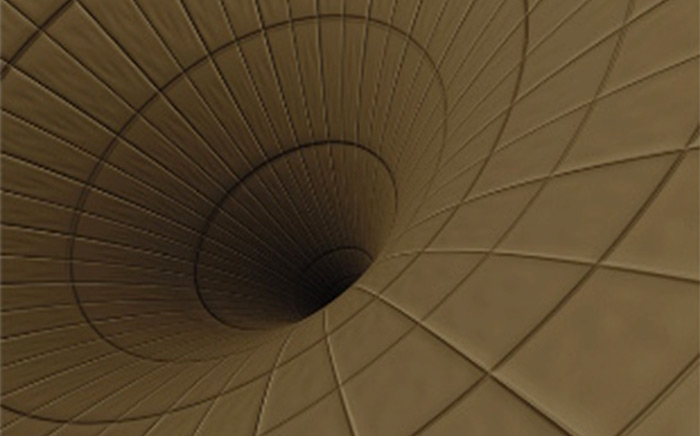# General RelativityThe general theory of relativity is Einstein's theory of gravity. Here he shows that gravity should not be described as a force, as in Newtonian physics, but instead as a consequence of the curvature of space and time. In short, gravity is spacetime geometry.

This course is about this remarkable discovery: what it means, how the theory can be (and has been) tested, its predictions and consequences.

## Course material

(Will be updated during the course.)

## Course content

We start by introducing the basic ideas behind the theory, such as the equivalence principle, how to describe curved spaces and spacetimes in terms of a metric, and the true importance of special relativity as a locally valid theory. Then we use this to study the most important case: the Schwarzschild spacetime, that is, the spacetime outside a spherically symmetric mass distribution such as a planet, a star or a black hole.

Only thereafter we move on to the more mathematical parts of the theory, introducing covariant derivative, the curvature tensor, the stress energy tensor and Einstein's equations.

We then discuss some interesting applications of the theory, such as black holes, cosmology and gravitational radiation.

## Course literature

James B. Hartle: Gravity - an Introduction to Einstein's General Relativity (Addison Wesley 2003, ISBN: 0-8053-8662-9)

Note that the book now exists in two versions, the original one and, unfortunately, a bad new one.

This is the one you should buy:

Do NOT buy the so called "New international edition" from Pearson: